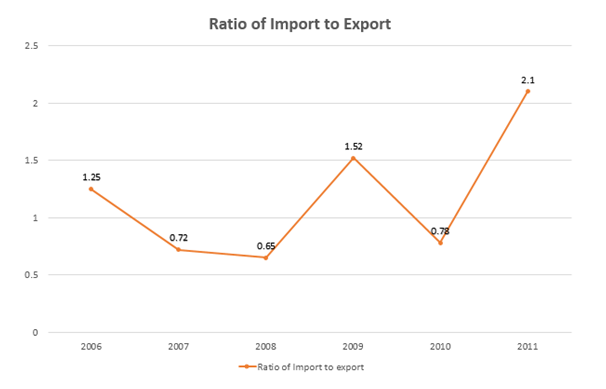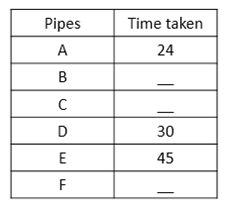# Quantitative Aptitude Questions (Data Interpretation) for SBI Clerk 2018 Day-24

## Quantitative Aptitude Questions (Data Interpretation) for SBI Clerk 2018 Day-24:

Dear Readers, SBI is conducting Online preliminary Examination for the recruitment of Clerical Cadre. preliminary Examination of SBI Clerk was scheduled from March 2018. To enrich your preparation here we have providing new series of Data Interpretation – Quantitative Aptitude Questions. Candidates those who are appearing in SBI Clerk Prelims Exam can practice these Quantitative Aptitude average questions daily and make your preparation effective.

Click “Start Quiz” to attend these Questions and view Solutions

## Daily Practice Test Schedule | Good Luck

 Topic Daily Publishing Time Daily News Papers & Editorials 8.00 AM Current Affairs Quiz 9.00 AM Logical Reasoning 10.00 AM Quantitative Aptitude “20-20” 11.00 AM Vocabulary (Based on The Hindu) 12.00 PM Static GK Quiz 1.00 PM English Language “20-20” 2.00 PM Banking Awareness Quiz 3.00 PM Reasoning Puzzles & Seating 4.00 PM Daily Current Affairs Updates 5.00 PM Data Interpretation / Application Sums (Topic Wise) 6.00 PM Reasoning Ability “20-20” 7.00 PM English Language (New Pattern Questions) 8.00 PM General / Financial Awareness Quiz 9.00 PM

Direction: (Q. 1 – 5) Study the following information carefully and answer the questions given below:

The following line graph represents the ratio of import to export of a company in six different years.1. If the import of the company in 2008 is 3.25 lakh and sum of the exports of the company in 2008 and 2009 together is 13.8 lakh, What is the import of the company in 2009?
1. 13.736 lakh
2. 15.376 lakh
3. 13.376 lakh
4. 15.376 lakh
5. none of these
1. What is the percentage increase in export from the year 2006 to 2009?
1. 21.6
2. 2.16
3. 16.2
5. none of these
1. The import of the company in 2008 is 11.7 and the export of the company in 2010 is same as the import in 2008. Then the export of the company in 2008 is approximately what percentage of the import of the company in 2010?
1. 158.235
2. 168.238
3. 197.238
4. 185.235
5. none of these
1. If in both the years 2006 and 2011 the import is 5.6 lakh, What is the sum of exports of the company in both the years?
1. 7.15 lakh
2. 8.15 lakh
3. 7.65 lakh
4. 8.25 lakh
5. none of these
1. If the export of the company in 2007 is 7.6 lakh, what is the import of the company in 2007?
1. 4.652 lakh
2. 5.472 lakh
3. 5.247 lakh
4. 2.248 lakh
5. none of these

Direction: (Q. 6 – 10) Study the following information carefully and answer the questions given below:

The following table represents time taken(in hours) by different pipes to fill a cistern. Some values are missing.1. If A and C kept open for 4 hours then A is replaced by D and kept open for 5 more hours, the tank is filled. In how many hours pipe C alone can fill the cistern?
1. 27/4 hours
2. 27/2 hours
3. 25/4 hours
4. 23/2 hours
5. none of these
1. Two pipes D and E are opened simultaneously to fill the cistern. After how much time should D be closed so that E alone can fill the cistern in another 20 hours?
1. 8 hours
2. 14 hours
3. 12 hours
4. 10 hours
5. none of these
1. If C takes half of the time taken by F to fill the cistern and F takes half of the time taken by B to fill the cistern and all of them working together can fill the cistern in 48 hours, What is the time taken by F to fill the cistern?
1. 152 hours
2. 142 hours
3. 186 hours
4. 168 hours
5. none of these
1. Two pipes A and D can fill the cistern. If they are opened on alternative hours and if pipe A is opened first, in how many hours will the cistern be full?
1. 24(3/5) hours
2. 26(3/5) hours
3. 26(3/4) hours
4. 25(3/4) hours
5. none of these
1. If B can fill the tank in 12 hours and pipe A works with one third of its efficiency, In how much time both the pipes can fill the cistern?
1. 10 2/7 hours
2. 7 2/7 hours
3. 5 3/7 hours
4. 12 4/7 hours
5. none of these

Direction: (Q. 1 – 5)

According to the question:

3.25/export(2008) = 0.65

=> export(2008) = 3.25/0.65

=>export(2008) = 5 lakh

Now, Export(2009) = 13.8 – 5 = 8.8 lakh

Import(2009)/8.8 = 1.52

=> import(2009) = 13.376 lakh

Hence, import of the company in 2009 is 13.376 lakh.

In the above line graph ratio of import to export is given and from this information we cannot calculate the values of export of the companies. Hence given data is not adequate to solve the question.

The import of the company in 2008 is 11.7

The ratio of import/export in the company 2008 =65/100

11.7/export(2008) = 0.65

Export(2008) = 18

The ratio of import/export in the company 2010 =78/100

Import(2010)/ 11.7 = 0.78

Import(2010) = 9.126

Required Percentage= (18/9.126)*100 =197.238

according to the question

5.6/export(2006) = 1.25

=> export(2006) = 5.6/1.25

=> export(2006) = 4.48 lakh

And

5.6/export(2011) = 2.1

=> export(2011) = 5.6/2.1 = 2.67 lakh

Required sum = 4.48 + 2.67 = 7.15 lakh

according to the question:

import(2007)/7.6 = 0.72

=> import(2007) = 0.72 x 7.6

=> import(2007) = 5.472 lakh

Hence, import of the company in 2007 is 5.472 lakh

Direction: (Q. 6 – 10)

let C takes X hours to fill the cistern.

According to the question:

4/24 + 4/x + 5/x + 5/30 = 1

=> 1/6 + 9/x + 1/6 = 1

=> 2/6 + 9/x = 1

=> 1/3 + 9/x = 1

=> 9/x = 1-1/3

=> 9/x = 2/3

=> x = 27/2 hours

Hence, C can alone fill the cistern in 27/2 hours.

let D should be closed after x hours.

x/30 + x/45 + 20/45 = 1

=> (3x+2x)/90 = 1-20/45

=> 5x/90 = (45-20)/45

=> x/18 = 25/45

=> x = 10 hours.

Hence, D should be closed after 10 hours.

let time taken by B to fill the cistern = 4x hours

hence, time taken by F to fill the cistern = 2x hours

and time taken by C to fill the cistern = x hours

according to the question:

48/x + 48/2x + 48/4x = 1

=> (192 + 96 + 48)/4x = 1

=> 4x = 336

=> x = 336/4

=> x = 84 hours.

Hence, time taken by F to fill the cistern = 2 x 84 = 168 hours.

part of the tank filled in 2 hours = 1/24 + 1/30 = 3/40

=>part of the tank filled in 2 x 13 hours = 3 x 13/40 = 39/40

Remaining part = 1 – 39/40 = 1/40

Since A is opened first, time taken by A to fill 1/40 part:

x/24 = 1/40

=> x = 3/5

Hence total time = 2 x 13 + 3/5 = 26(3/5) hours.

let both the pipes can fill the cistern in x hours.

According to the question:

x/12 + x/(24 * 3) = 1

=> x/12 + x/72= 1

=> (6x + x)/72 = 1

=> 7/72= 1

=> x = 10 2/7 hours.

Hence, both the pipes can fill the cistern in 10 2/7 hours.